### Home > PC > Chapter 1 > Lesson 1.4.2 > Problem1-149

1-149.
1. Given f(x) =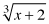. Homework Help ✎

1. Find f −1(x).

2. Sketch f(x) and f1(x) on the same set of axes.

3. Find f1(f(6)) and f(f −1(2)). What do you notice?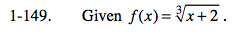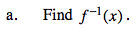Change f(x) to y.
Switch every x to y and every y to x.
Solve for y.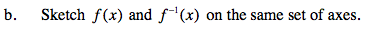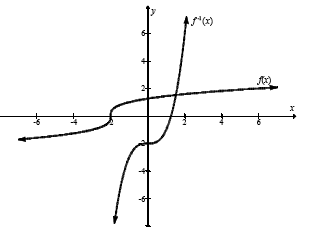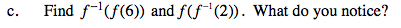Composing f and f −1 in either order returns the original number.